## Graphical Axioms from the nCatLab

If you don’t read the n-category café’s wiki project, you are missing out.

Definition. A Frobenius algebra in a monoidal category is a quadruple (A,δ,ϵ,μ,η) such that

1. (A,μ,η) is a monoid,
2. (A,ϵ) is a comonoid, and
3. the Frobenius laws hold: (1⊗μ)∘⊗1)=δ∘μ=(μ⊗1)∘(1δ).

In terms of string diagrams, this definition says:The first line here shows the associative law and left/right unit laws for a monoid. The second line shows the coassociative law and left/right counit laws for a comonoid. The third line shows the Frobenius laws.

Certain kinds of Frobenius algebras have nice PROPs or PROs. The PRO for Frobenius algebras is the monoidal category of planar thick tangles, as noted by Aaron Lauda Lauda (2006) and illustrated here:Lauda and Pfeiffer Lauda (2008) showed that the PROP for symmetric Frobenius algebras is the category of ‘topological open strings’, since it obeys this extra axiom: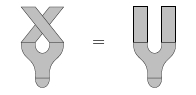… [A]ny commutative Frobenius algebra gives a 2d TQFT…. The monoid laws look like this: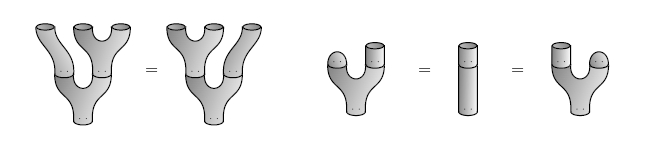The comonoid laws look like this: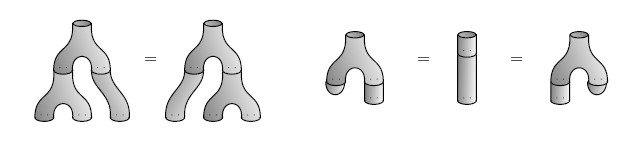The Frobenius laws look like this: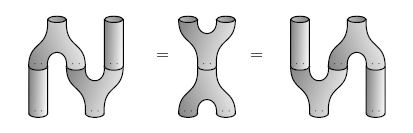and the commutative law looks like this: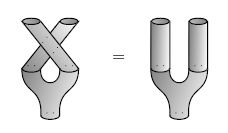A special commutative Frobenius algebra gives a 2d TQFT that is insensitive to the genus of a 2-manifold, since in terms of pictures, the ‘specialness’ axioms ∘δ=1 says that